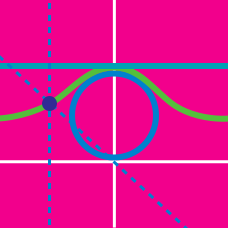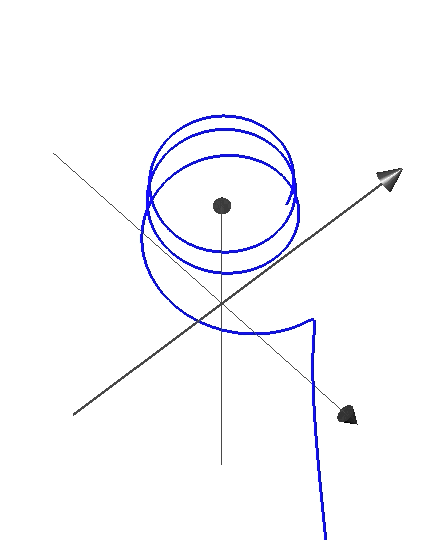Algebra

# Parametric Equations: Level 2 Challenges

The curve described parametrically by $\begin{cases} x=t^{2}+t \\ y=t^{2}-t \end{cases}$ represents which of the following shapes?The parametric equations for the curve shown are $\begin{cases} x = \cos(t) \\ y = \sin(t) \\ z = \text{?} \end{cases}$ where $t$ ranges over $(0, 20)$. Which function could $z$ be equal to?

$\begin{cases} x = e^t \\ y = e^{2t} - 1 \end{cases}$

What is the shape of the curve described by the above parametric equation?

A point $\left( x,y \right)$ moves counterclockwise along the unit circle at constant angular speed $\omega$. Describe the motion of the point $\left( -2xy, { y }^{ 2 }-{ x }^{ 2 } \right)$.

Two clowns, Twinkle and Jingle, are throwing pies at each other. Twinkle throws a pie toward Jingle from $500$ centimeters away. Its flight path is given by parametric equations $\begin{cases} x &=& 100t \\ y &=& 80t - 16t^2 \end{cases}$ where $t$ is time in seconds.

Two seconds later Jingle launches an interceptor pie from his location with the flight path $\begin{cases} x &=& 500 - 500(t-2) \\ y &=& K(t-2) - 16(t-2)^2 \end{cases}$ Find the value of $K$ which will guarantee that the interceptor pie will hit its target (the pie thrown by Twinkle).

×